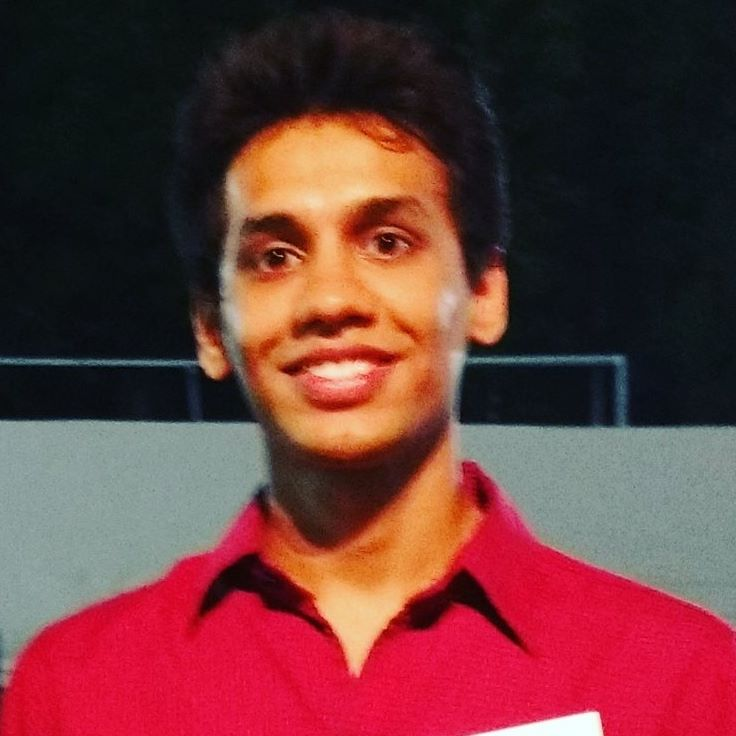# Longest Substring Unique CharactersQueue implementation to find the longest substring inside a string with non-repeating characters.

Time Complexity: O(N)

public int lengthOfLongestSubstring(String s) {
int maxLength = 0;

if(s.length() == 0)
return maxLength;
if(s.length() == 1)
return 1;

int endIndex = 0;

//Keep adding distinct characters to queue

while(endIndex < s.length()){
Character next = s.charAt(endIndex);
//if the character on next index is already present in queue -> poll the queue
//until you find the character
if(subLong.contains(next)){
while(true){
Character curr = subLong.poll();
System.out.println("popping: " + curr);
if(curr == next){
break;
}
}
}
//every time keep adding in queue and check the size of queue with maxLength